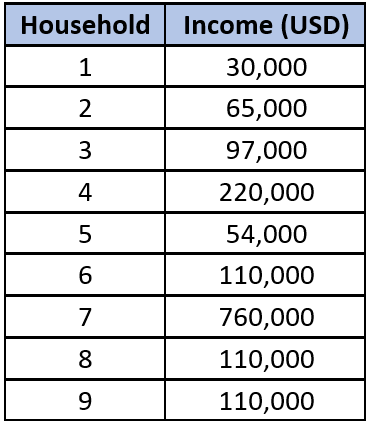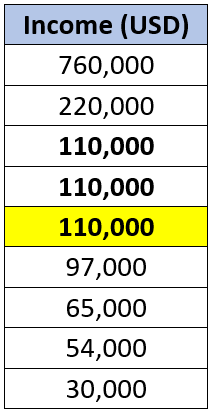## Interpreting a Dataset

Determine the median, mode, and mean for the data set of household incomes.Hint
The mean is the average of all the data points. The median is the value separating the higher half from the lower half of the data set. The mode is the value that occurs most often.
Hint 2The mean is the average of the nine data points:
$$mean=\frac{sum\:of\:the\:terms}{number\:of\:terms}=\frac{1,556,000\:USD}{9}=172,889\:USD$$$When the discrete data are rearranged in increasing order and the number of terms, $$n$$ , is odd, the median is the value of the $$(\frac{n+1}{2})^{th}$$ item. When $$n$$ is even, the median is the average of the $$(\frac{n}{2})^{th}$$ and $$(\frac{n}{2}+1)^{th}$$ items. There are 9 items in this dataset, so the median will be the 5th item. After sorting the values in descending/ascending order,$110,000 is the middle value.The mode is the value that occurs the most often. After sorting the list by descending/ascending order, we find $110,000 is also the value that appears the most with three occurrences. The mean is$172,889.
The median is $110,000. The mode is$110,000.